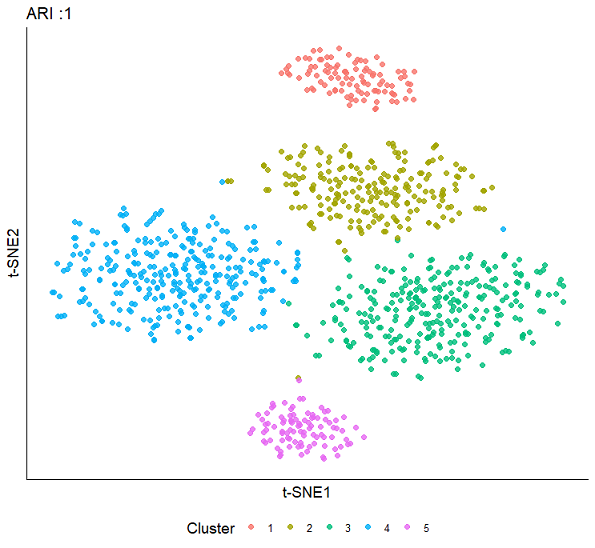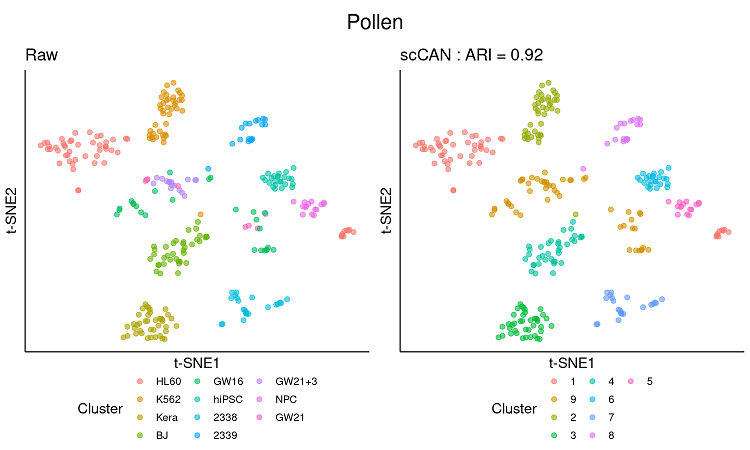# Introduction

Clustering is the key step to define cell types from the heterogeneous cells population. One critical challenge is that single-cell RNA sequencing (scRNA-Seq) experiments generate a massive amount of data with an excessive noise level, which hinders many computational algorithms. We propose a single cell Clustering method using Autoencoder and Network fusion (scCAN) that can accurately segregate cells from the high-dimensional noisy scRNA-Seq data. The main contributions of scCAN are: (1) correctly estimates the number of true cell types, (2) accurately segregates cells of different types, (3) is robust against dropouts, and (4) is fast and memory efficient. The scCAN package is available at https://cran.r-project.org/package=scCAN. Data and R scripts are available at http://sccan.tinnguyen-lab.com/.

# Preliminaries

This vignette gives step-by-step instruction for installing scCAN R package, clustering sample and real datasets, and validating clustering results with biological prior knownledge.

Install scCAN package from CRAN repository.

``install.packages("scCAN")``

Install package from source file.

``install.packages("scCAN_1.0.2.tar", repos = NULL)``

To start the analysis, load scCAN package:

``library(scCAN)``
``## Loading required package: scDHA``
``## Loading required package: FNN``

# Analysis on the sample dataset

scCAN takes input of expression matrix from single-cell RNA sequencing in which rows represent samples and columns represent genes. scCAN assumes the input has been previously normalized by the users. The only pre-processing step that scCAN performs is to rescale the expression data to a range from 0 to 1 for each cell to reduce the technical variability and heterogeneous calibration from sequencing technologies. Install pakcage from CRAN. scCAN package includes a sample dataset of 1,000 cells and 5,000 genes for a quick demonstration.

``````#Load example data (SCE dataset)
data("SCE")
#Get data matrix and label
data <- t(SCE\$data); label <- as.character(SCE\$cell_type1)``````

Inspect a sample of 10 cells and 10 genes.

``data[1:10,1:10]``
``````##        [,1] [,2] [,3] [,4] [,5] [,6] [,7] [,8] [,9] [,10]
## Cell1    34   30    0    4    0   16    7   13  126     0
## Cell2    59   32    0   15    6   22    0   23  136     0
## Cell3    12   27    0    8    2    6   13   14  114     0
## Cell4    27   17    0    4    0    1    1    4   58     0
## Cell5    15   25    5    0    0    5   20   27  131     0
## Cell6    20    3    0    3    0    0    0    7   67     0
## Cell7    19    7    0    0    4    0    3   12   36     0
## Cell8    65   10    0    3    1    5   13   21  127     0
## Cell9    34    0    3    5    1    8    8   13   83     0
## Cell10   41   17    0    0    0    0   14   26  164     0``````
``dim(data)``
``##  1000 5000``

The expression matrix should represent cells by rows and genes by columns.

Now, we can view the of annotations of 1,000 cells that belong to 5 separate groups.

``label[1:10]``
``##   "2" "4" "3" "4" "1" "3" "3" "3" "4" "3"``
``print(paste0("The number of cell types : ",length(unique(label))))``
``##  "The number of cell types : 5"``

The annotation file could be stored in a vector in which each element is a cell type label of each cell.

Next, we can cluster the sample dataset using the following command.

``````#Generate clustering result, the input matrix has rows as samples and columns as genes
result <- scCAN(data)
#The clustering result can be found here
cluster <- result\$cluster
#Calculate adjusted Rand Index using mclust package
print(paste0("ARI = ", ari))``````

The next step is to visualize the expression data with obtained clusters. We use irlba  R package to quickly reduce the original data dimension to 20, and we use Rtsne  package to obtain 2D embedding data. Finally, we can visualize the cells landscape using scatter plot available in ggplot2  package.

``````suppressPackageStartupMessages({
library(irlba)
library(ggplot2)
library(Rtsne)
})
# Get 2D emebedding data of the original data.
set.seed(1)
low <- irlba::irlba(data, nv = 20)\$u
tsne <- as.data.frame(Rtsne::Rtsne(low)\$Y)
tsne\$cluster <- as.character(cluster)
colnames(tsne) <- c("t-SNE1","t-SNE2","Cluster")
p <- ggplot2::ggplot(tsne, aes(x = `t-SNE1`, y = `t-SNE2`, colour = `Cluster`))+
ggtitle(paste0("ARI :", ari))+labs(color='Cluster')+
geom_point(size=2, alpha = 0.8) +
theme_classic()+
theme(axis.text.x=element_blank(),
axis.ticks.x=element_blank(),
axis.text.y=element_blank(),
axis.ticks.y=element_blank(),
axis.title=element_text(size=14),
plot.title = element_text(size=16),
panel.grid.major = element_blank(),
panel.grid.minor = element_blank(),
legend.position="bottom", legend.box = "horizontal",
legend.text =element_text(size=10),
legend.title=element_text(size=14))+
guides(colour=guide_legend(nrow = 1))``````Visualization of expression data using cells clusters identified from scCAN.

# Analysis on real human tissue dataset

We will use a real dataset from  that has 301 cells sequenced from human tissues including blood, skin, brain, and stem cells.

``````suppressPackageStartupMessages({
library(SummarizedExperiment)
})
path <- "https://bioinformatics.cse.unr.edu/software/scCAN/data/pollen.rds"
# Get expression matrix
data <- t(SummarizedExperiment::assay(SCE))``````
``## Loading required package: SingleCellExperiment``
``````# Get cell annotation
label <- SummarizedExperiment::colData(SCE)``````

Check the expression matrix.

``data[1:10, 1:10]``
``````##             A1BG A1BG-AS1 A1CF A2LD1 A2M A2M-AS1 A2ML1 A2MP1 A4GALT A4GNT
## Hi_2338_1   9.08     0.00 0.00  0.00   0       0  0.10     0   0.57     0
## Hi_2338_2   0.00     0.00 0.05  0.00   0       0  0.00     0   0.00     0
## Hi_2338_3   0.00     3.47 0.00  0.00   0       0  0.14     0   0.00     0
## Hi_2338_4   1.75     0.36 0.00  0.29   0       0  0.00     0   0.00     0
## Hi_2338_5   0.00     0.00 0.00  0.00   0       0  0.00     0   0.00     0
## Hi_2338_6   0.40     0.00 0.00  9.19   0       0  0.00     0   0.00     0
## Hi_2338_7   0.00     0.00 0.00  0.00   0       0  0.00     0   0.35     0
## Hi_2338_8   0.78     0.00 0.00  0.00   0       0  0.00     0   0.00     0
## Hi_2338_9  18.74     0.00 0.00  0.00   0       0  0.28     0   0.00     0
## Hi_2338_10  0.32     0.00 0.00  0.00   0       0  0.00     0   0.00     0``````
``dim(data)``
``##    301 23730``

Check the annotation file.

``head(label)``
``````## DataFrame with 6 rows and 2 columns
##           cell_type1 cell_type2
##             <factor>   <factor>
## Hi_2338_1       2338     dermal
## Hi_2338_2       2338     dermal
## Hi_2338_3       2338     dermal
## Hi_2338_4       2338     dermal
## Hi_2338_5       2338     dermal
## Hi_2338_6       2338     dermal``````

The annotation file is stored data frame in which rows are samples. The annotation file has two sets of labels: (i) cell_type1 indicates cell types discovered from the authors, (ii) cell_type2 has annotation of tissues that the cells was taken. In our annlysis, we use cell_type1 for validation and visualization.

Next, we can cluster the sample dataset using the following command.

``````#Generate clustering result, the input matrix has rows as samples and columns as genes
start <- Sys.time()
result <- scCAN(data)
running_time <- difftime(end,start,units = "mins")
#The clustering result can be found here
cluster <- cluster\$cluster
#Calculate adjusted Rand Index using mclust package
print(paste0("ARI = ", ari))
print(paste0("Running time = ", running_time))``````

We use the same approach mentioned above to obtain 2D embedding data of Pollen dataset. The visualization of original data using true labels and scCAN’s clusters are shown below:Visualization of expression data using original cell types and cluster identified from scCAN for Pollen dataset.

scCAN was able to cluster Pollen dataset in 1 minutes with 9 clusters identified. The clusters discovered by scCAN are highly correlated with cell labels obtained from the original publication (ARI = 0.92).

## Visualizing latent data

scCAN uses two autoencoders to generate compressed representation of original expression data. In our model, we limit the size of the latent layer to a low number of dimensions (d = 15 by default). We can always visualize the latent data using standard dimensional reduction algorithms including t-SNE  and UMAP .

``````suppressPackageStartupMessages({
library(Rtsne)
library(umap)
})
# Get latent data from scCAN result
latent <- result\$latent
# Generating 2D embedding data using t-SNE and UMAP
set.seed(1)
tsne.data <- Rtsne(latent, check_duplicates = F,dims = 2)\$Y
umap.data <- umap(latent,n_components = 2)\$layout``````

The 2D visualizations of the latent data are shown as follows: